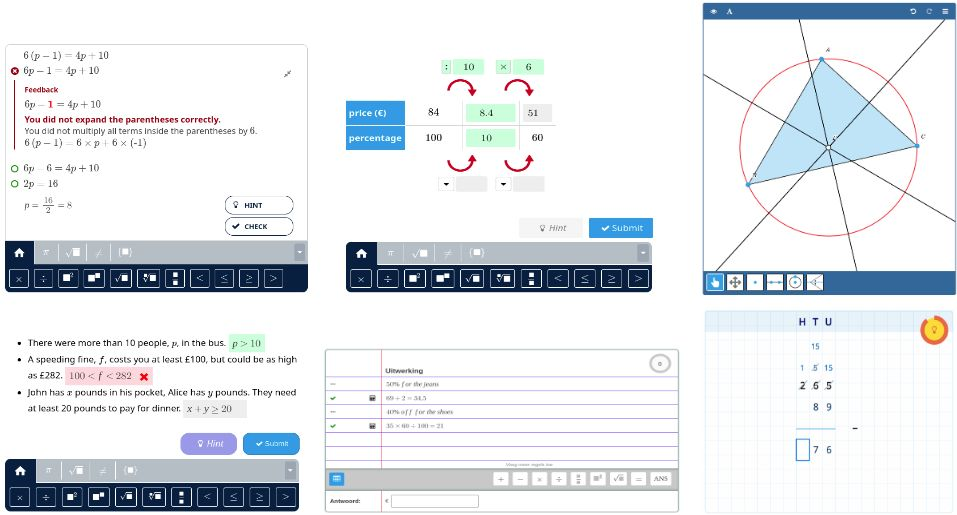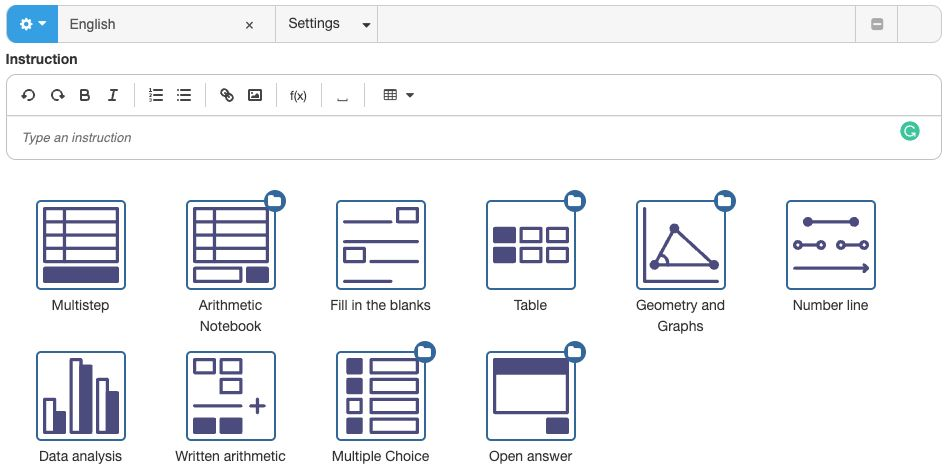# Main Components

## Overview

In this tutorial, we will discuss the following components of the Algebrakit system:

• Question types are the interactive components that run on the web page and allow students to work on math questions.
• The Authoring component and Algebrakit CMS allow you to create custom Algebrakit questions.
• The Formula editor is the tool to conveniently write mathematical expressions.

## Question types

We offer a question type for each type of math activity. Some of the most-used question types are:

• Multistep allows students to solve problems in steps by writing math expressions. This question type applies to various math topics, such as algebra, equations, and calculus.
• Fill in the blanks offers input fields within a paragraph.
• Math Table applies to mathematical tables, which you can use in many situations. Examples are function tables, ratio tables, recurrence relations, etc.
• Geometry & Graphs applies to two-dimensional geometry, coordinates, and function graphs.
• Arithmetic Notebook is a question type for arithmetic story problems.
• Written Arithmetic applies to column-based arithmetic as used in primary education.Figure: The question types Multistep, Math Table, Geometry & Graphs, Fill in the Blanks, Arithmetic Notebook, and Written Arithmetic

## Authoring component and Algebrakit CMS

There are two ways to create new Algebrakit questions.

1. The Algebrakit Content Management System (CMS) is a web-based application that allows you to create Algebrakit content. The Algebrakit CMS is built and maintained by the Algebrakit team. Exercises created will be stored within the Algebrakit CMS and can be referred to through a unique identifier (the "exercise ID").
2. The Authoring component is a tool that exists within another content management application. You can create and store the exercises directly from the host application, so there is no need to work with exercise IDs.

Depending on how Algebrakit is integrated into your software environment, you work with either the Algebrakit CMS or the Algebrakit Authoring component. In both cases, the details of creating Algebrakit questions will be similar.

If you don't have access to either of these authoring options, then you can use the Testbench to experiment with Algebrakit.Figure: The Algebrakit Authoring tool